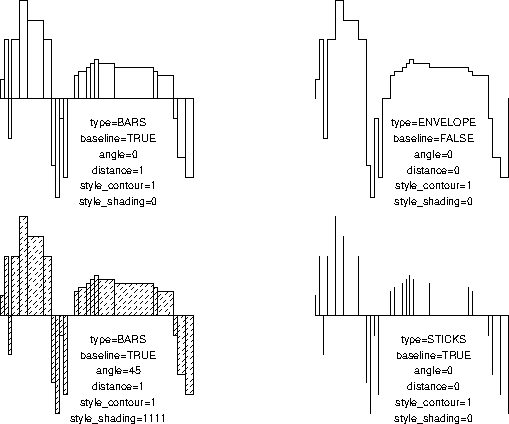# plhiss

parametertypeunitsdescription
x,yfloat*uuarrays defining the histogram
nint--number of elements in the arrays
typeint--histogram type: BARS, ENVELOPE or STICKS
baselineint--if TRUE, a baseline is drawn
ybasefloatuuy-position of the baseline, if any
style_contourint--line style for the histogram contours
anglefloatdegrangle for hatching
distancefloatmmdistance between hatching lines
returns:void

## Description

plhiss plots a histogram using values in arrays x (position) and y (height+ybase). x must be in ascending order. If baseline is TRUE a baseline is drawn at y=ybase. type determines the histogram type and can have one of three predefined values: BARS, ENVELOPE and STICKS. See the example for further explanation. The histogram contours are dashed according to the parameter style_contour the histogram may also be hatched by setting distance to a non-zero value.

## Examples

```#include <simplot.h>
int main() {
float x[] = { 1, 2, 3, 4, 6, 8,12,14,15,16,17,18,20,
21,23,24,25,26,30,40,41,45,46,48,50};
float y[] = { 5,15,-10,15,25,20,15,-17,-25,-5,-20,0,
6,7,8,9,10,9,8,7,6,-5,-15,-20,-25};
int type[] = {BARS, BARS,  ENVELOPE, STICKS};
int base[] = {TRUE, TRUE,  FALSE,    TRUE};
int das[]  = {DOWN, DOWN,  DOWN,     DOWN};
int ang[]  = {0,    45,    0,        0};
int dis[]  = {1,    1,     0,        0};
int sda[]  = {0,    1111,  0,        0};
char *s={"FALSE","TRUE","STICKS","ENVELOPE","BARS"};
int i;

plinit(PS,"plhiss",200,150,0,0,"","");
for (i=0;i<4;i++) {
plreserv(30,25,55);
plhiss(x,y,25,type[i],base[i],0,das[i],ang[i],dis[i],sda[i]);
plot(31,-5,UP);
plformat(0,-.5,"type=%s\n"
"baseline=%s\n"
"angle=%d\n"
"distance=%d\n"
"style__contour=%d\n"
s[type[i]],s[base[i]],ang[i],dis[i],das[i],sda[i]);
}
exit(0);
}
```• Home